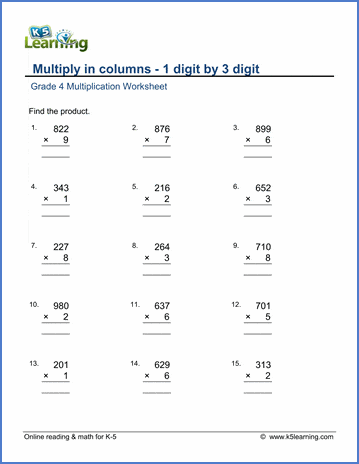# Multiply in Columns Worksheets for Grade 4

## Multiplication worksheets

Multiplying numbers in columns is a math skill which requires lots of practice. Our grade 4 multiplication in columns worksheets range in difficulty from 2 x 1 digits to 3 x 3 digits. These math worksheets complement our grade 4 mental multiplication worksheets which focus on practicing "in your head" multiplication skills.

## Multiply in Columns

2-digit by 1-digit 12
X 4

3-digit by 1-digit 123
X 4

4-digit by 1-digit 1,234
X 4

## Double digit multiplication

2-digit by 2-digit 12
X 12

3-digit by 2-digit 123
X 45

4-digit by 2-digit

1,234
X 45

3-digit by 3-digit 123
X 123

"Mental" multiplication 4 X 105 =Sample grade 4 multiplication in columns worksheet

## More multiplication worksheets

Find all of our multiplication worksheets, from basic multiplication facts to multiplying multi-digit whole numbers in columns.

What is K5?

K5 Learning offers free worksheets, flashcards and inexpensive workbooks for kids in kindergarten to grade 5. Become a member to access additional content and skip ads.